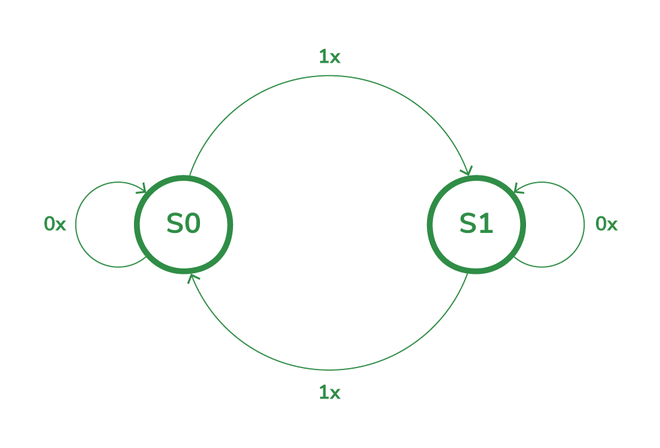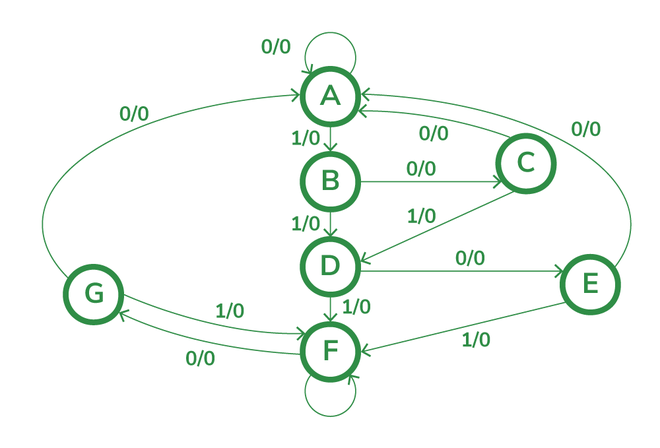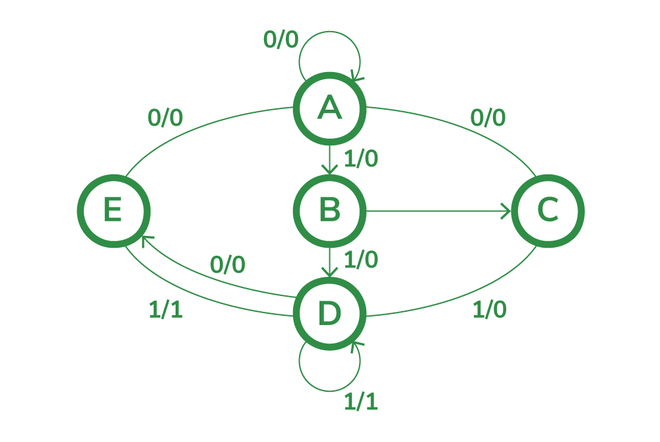# State Reduction and State Assignment

• Last Updated : 04 Jul, 2022

To illustrate the process of state reduction and state assignment first we have to know the concepts of the state diagram, state table, and state equation. In this article, we are going to learn all the topics related to state reduction and assignment.

State diagram: The state graph or state diagram is a pictorial representation of the relationships between the present state, the input state, the next state, and the output state of a sequential circuit i.e. A state diagram is a graphical representation of a sequential circuit’s behavior.

Example: Consider an excitation table of J-K flip-flop

The state diagram of the above table isState Diagram of J-K flip-flop

State table: Even though the behavior of a sequential circuit can be conveniently described using a state diagram, for its implementation the information contained in the state diagram is to be translated into a state table. The tabular form of the state diagram is the state table. The present state, the next state, and the output are the three sections of the diagram.

The state table of JK flip-flop is:

State equation: Qn+1 = Qn bar J + Qn K bar

### State reduction:

The state reduction technique generally prevents the addition of duplicate states. The reduction in redundant states reduces the number of flip-flops and logic gates,  reducing the cost of the final circuit. Two states are said to be equivalent if every possible set of inputs generates exactly the same output and the same next state. When two states are equal, one of them can be eliminated without changing the input-output relationship. The state reduction algorithm is applied in the state table to reduce equivalent states.

### State assignment:

State assignment refers to the process of assigning binary values to the states of a sequential machine. The binary values should be given to the states in such a way that flip-flop input functions may be implemented with a minimum number of logic gates.

State assignment rules are as follows:

Rule 1: States having the same next state for a given input condition should have assignments that can be grouped into logically adjacent cells in a K-map.

Rule 2: States that are the next states of a single state should have assignments that can be grouped into logically adjacent cells in a K-map.

Example 1: To explain the concept of state reduction let us consider the state table as

The state diagram for the above state table isState diagram before reduction

Step1: First here we are supposed to identify two or more similar states in the next state and output state. In the above table if we observe states of e and g are having the same next state and output values for all combinations of input i.e. X=0 and X=1.

So eliminate the g state in the state table and where ever g is present replace it with e. Because e and g both are the same i.e. e=g.

Step 2: Again check if any two states have similar values or not. If any two states have the same next state and output then eliminate one state.

Here d and f are having the same next state value and output. So eliminate f and where ever f is present replace it with d. Because both are the same d=f

Step 3: Further observe if any similar states are present or not. The states c and e are having same next states but they are having different outputs. So we can not consider it a reduction state.State diagram After reduction

Step 4:  If you observed the state table, the states are represented by using the alphabet. We can not proceed further if we are having alphabets, so, assigning binary numbers to alphabets is called a state assignment.

To assign binary numbers to the state we have to consider the minimum number of bits.

The codes must contain n bits for a circuit with m states, where 2n >= m. In this case, each state requires 23 >=5=>3 bits to be represented. With three bits, there are eight possible combinations, of which five can be used to represent the states.

Step 5: Replacing the alphabets with binary numbers.

My Personal Notes arrow_drop_up